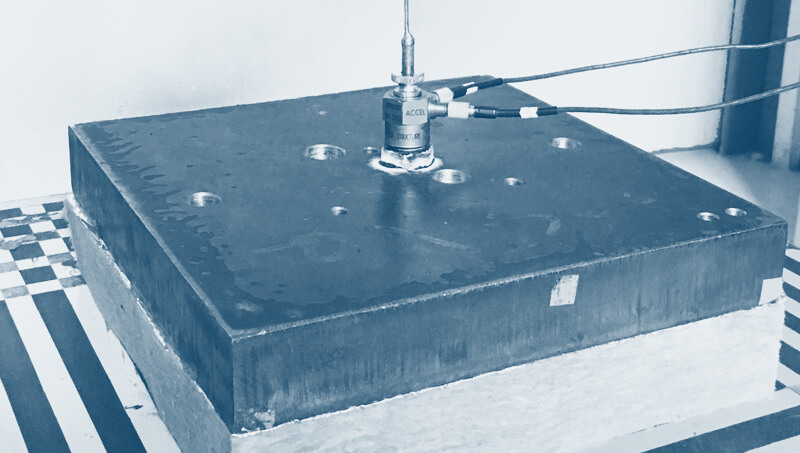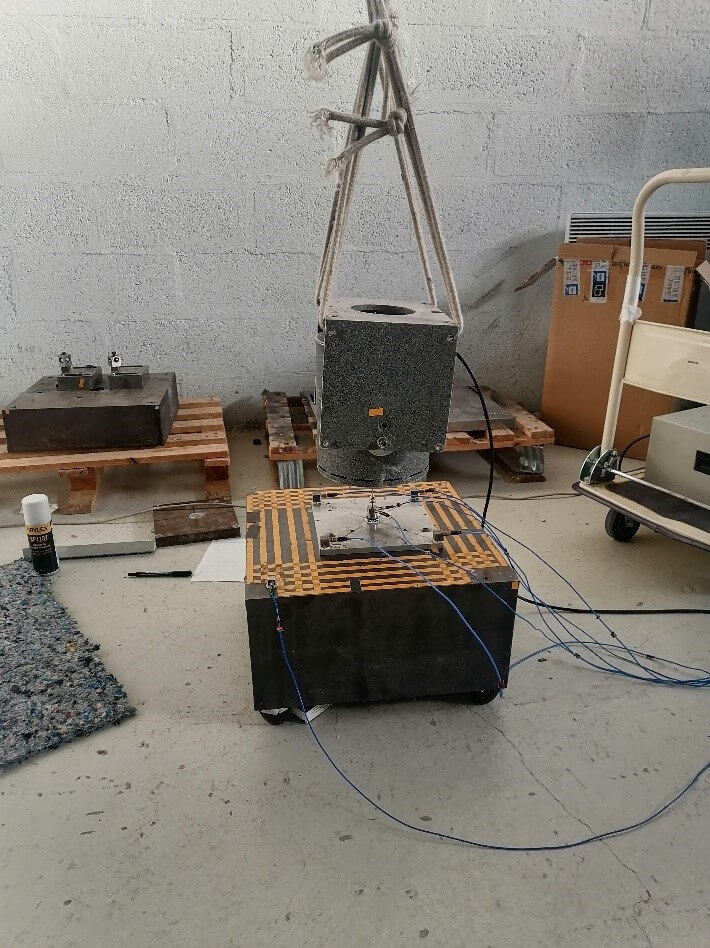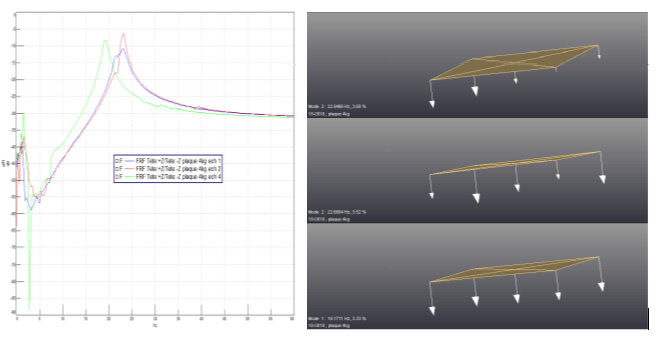Select Page

# Dynamic stiffness benchThe CEVAA has a dynamic stiffness bench to determine the elastic properties of a material.

Composition of equipment: Dynamic stiffness tests are carried out using a device based on the mass-spring-mass system. The sample is placed on a support mass of approximately 250 kg placed on decoupling pads. This sample is compressed using 1kg, 4kg or 8kg plates, on which is fixed an impedance head and 4 accelerometers. A vibrating pot normal to the sample allows the excitation of the whole.

Dimensions of specimens tested: Lx W = 20cm * 20cm

## Bench principles

The dynamic stiffness of a material is calculated from the resonance frequency obtained by plotting the frequency response function (FRF).

The loss factor of the sample is determined through a summary of the measured FRF. The modulus of elasticity is also calculated from the dynamic stiffness and the thickness of the sample.

#### Tests conditions

The measurements are carried out at ambient laboratory temperature. The impedance head and accelerometers are bonded with Loctite 409 glue.

#### Results

From the reading of the resonance frequency of the main peak, the calculations of dynamic stiffness, modulus of elasticity and loss factor are determined for each of the compression plates. The porosity of the material comes from the identification of a model of porous material from the acoustic measurements carried out.

The Figure shows the FRFs as well as the deformations obtained when the samples are compressed by a 4 kg plate.## MATERIALS CHARACTERIZATION BENCHES

Poisson's ratio characterization bench

Dynamic mechanical analysis (DMA)